Learning Library

These fun math puzzles are a great way for second graders to dig into math skills.

Click on a worksheet in the set below to see more info or download the PDF.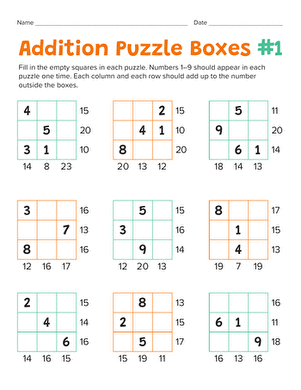Put your second grader's math skills to the test with mathematical puzzle boxes. He'll practice doing addition facts in his head, and build logic skills.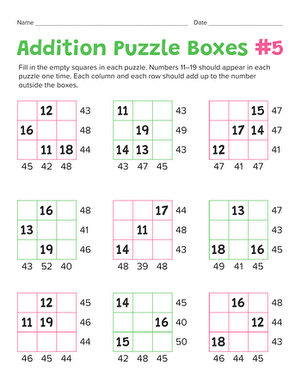Addition Math Puzzles #2

Your second grader will practice adding up to 50 while building her logic and problem solving skills with these math puzzle boxes.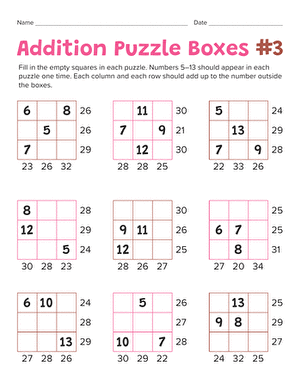Addition Math Puzzles #3

Put your child's addition abilities to the test with mathematical puzzle boxes. He'll practice his addition as he solves each puzzle.Addition Math Puzzles #4

Math lovers can put their addition and subtraction skills to the test to figure out these Sudoku-style puzzles, a perfect introduction to basic algebra!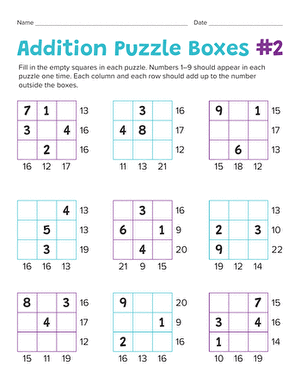Addition Math Puzzles #5

Make practicing math fun with math puzzles. Your child will learn the basics of solving logic problems as he does math in his head.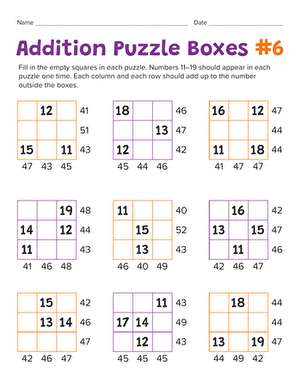Addition Math Puzzles #6

Add a fun twist to math practice with these addition puzzles. Your math maniac will get an introduction to logic and problem solving.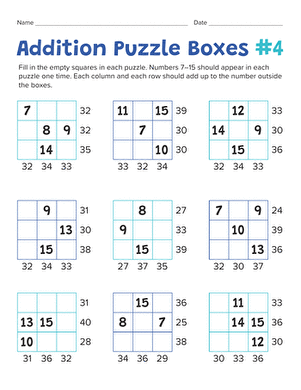Addition Math Puzzles #7

What better way to learn math than with an addition puzzle? Your math star will practice addition and get a taste of problem solving with logic.

Want to download this whole set as a single PDF?

Create new collection

0

New Collection>

0 items

What could we do to improve Education.com?

Please note: Use the Contact Us link at the bottom of our website for account-specific questions or issues.

What would make you love Education.com?